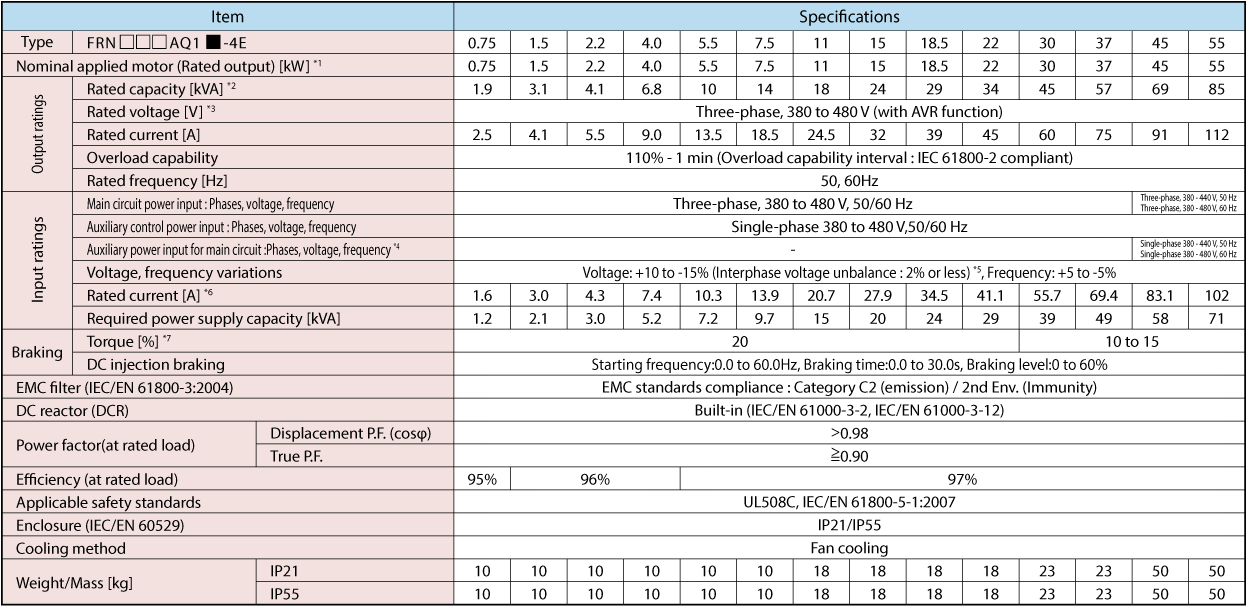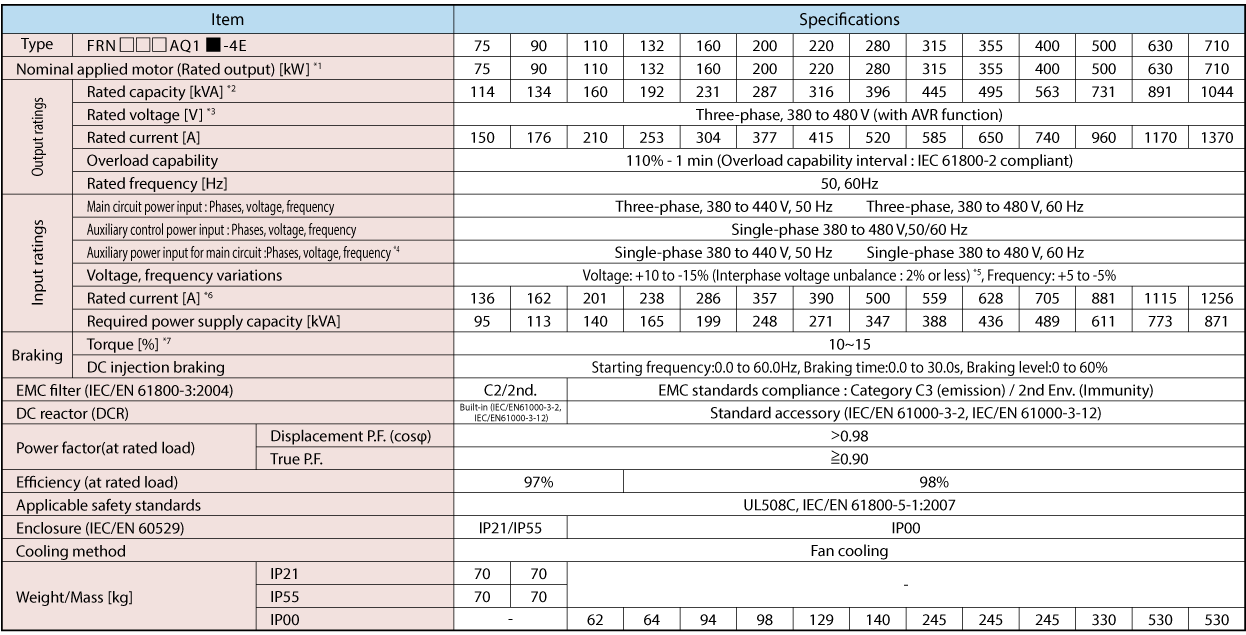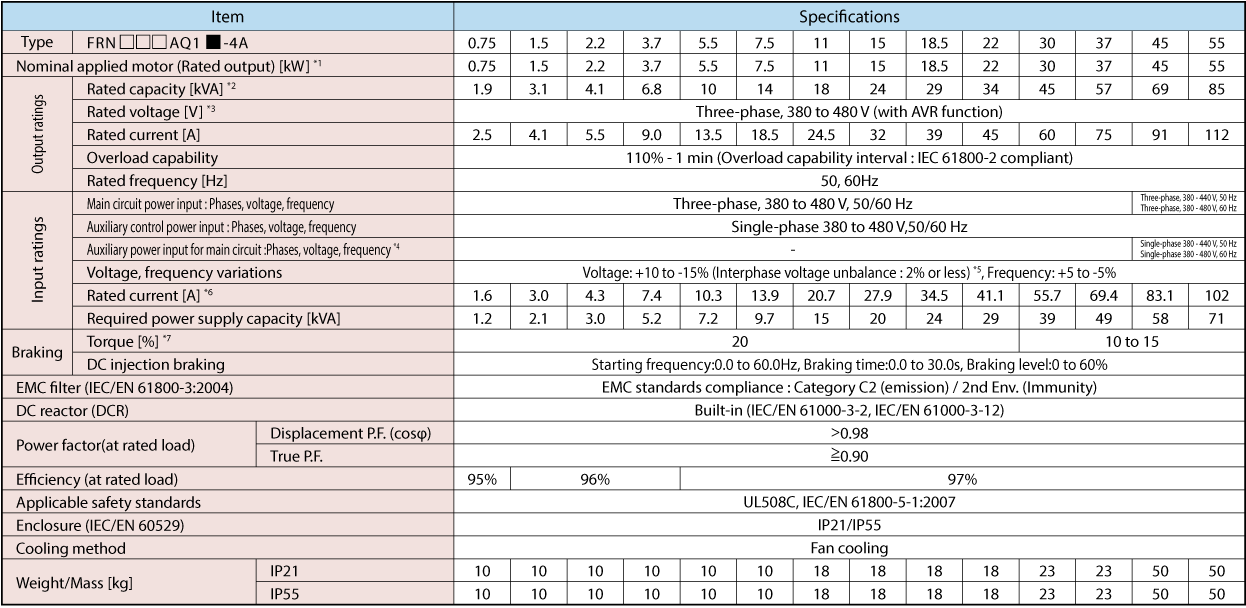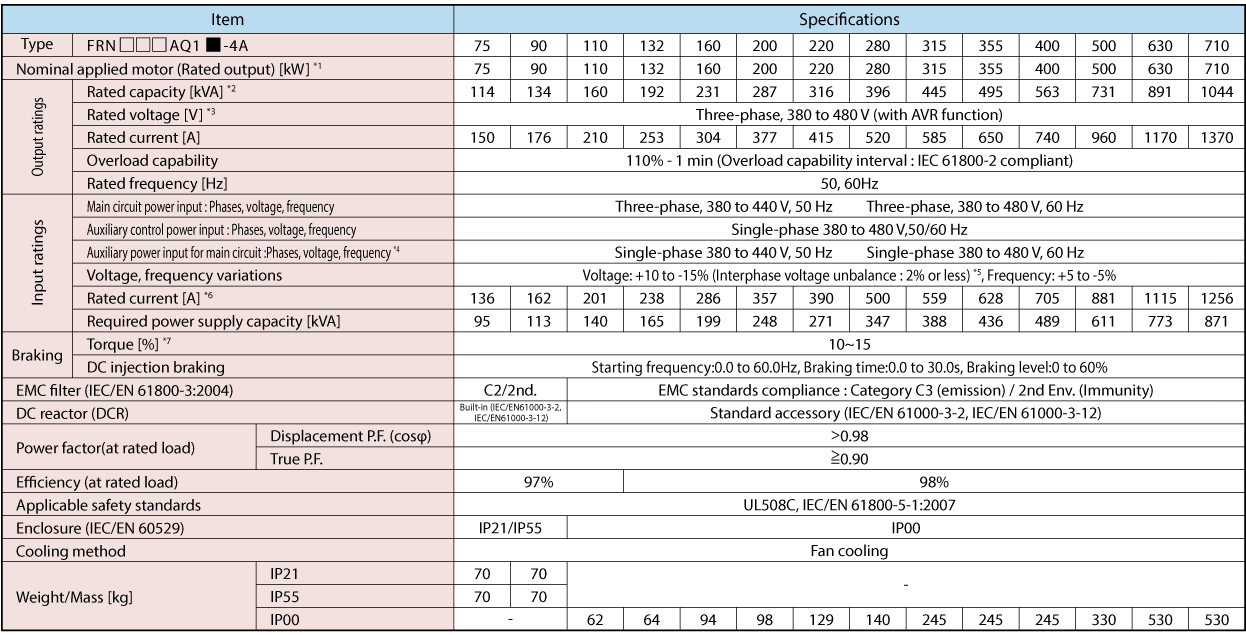Specifications

Specifications

Europe

3-phase, 400V series (0.75 to 55kW)3-phase, 400V series (75 to 710kW)*1) Fuji 4-pole standard motor.
*2) Rated capacity is calculated by assuming the output rated voltage as 440 V.
*3) Output voltage cannot exceed the power supply voltage.
*4) The auxiliary power input is used as an AC power input when combining the unit to DC power supply such as high power factor PWM converter with power regenerative function. (Generally not to be used.)
*5) Voltage unbalance [%] = (Max. voltage [V] - Min. voltage [V])/Three-phase average voltage [V] x 67 (See IEC61800-3.) If this value is 2 to 3%, use an optional AC reactor (ACR).
*6) The value is calculated on assumption that the inverter is connected with a power supply 400V, 50Hz and Rsce=120.
*7) Average braking torque for the motor running alone. (It varies with the efficiency of the motor.)

Asia

3-phase, 400V series (0.75 to 55kW)3-phase, 400V series (75 to 710kW)*1) Fuji 4-pole standard motor.
*2) Rated capacity is calculated by assuming the output rated voltage as 440 V.
*3) Output voltage cannot exceed the power supply voltage.
*4) The auxiliary power input is used as an AC power input when combining the unit to DC power supply such as high power factor PWM converter with power regenerative function. (Generally not to be used.)
*5) Voltage unbalance [%] = (Max. voltage [V] - Min. voltage [V])/Three-phase average voltage [V] x 67 (See IEC61800-3.) If this value is 2 to 3%, use an optional AC reactor (ACR).
*6) The value is calculated on assumption that the inverter is connected with a power supply 400V, 50Hz and Rsce=120.
*7) Average braking torque for the motor running alone. (It varies with the efficiency of the motor.)